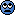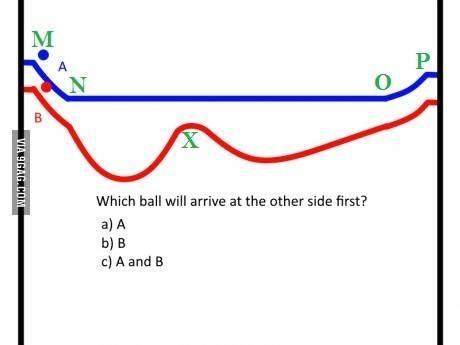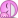# Physics riddle

• micromass

#### micromass

Staff Emeritus
Homework Helper
http://img-9gag-fun.9cache.com/photo/aX9zqD2_460s.jpg [Broken]

Last edited by a moderator:
•jedishrfu and QuantumQuest

B
NOT 2 B

•jedishrfu
Without specific data and judging solely from the diagram I intuitively tend to vote for B.

•jedishrfu
If the start and stop height differences are the same, wouldn't the answer be C?

•atom jana and jedishrfu
If the start and stop height differences are the same, wouldn't the answer be C?

HINT:

NOT 2 B

What was the question again?

If the lines are frictionless then it shouldn't matter

HINT:

Well, when you put it that way...•billy_joule
If the lines are frictionless then it shouldn't matter

Suppose the blue line had a smaller slope at the beginning and end. The ball could take a long time to get across.

And, if the red line had a really large dip, it could take a long time to get across.

I reckon the red will be behind at the top of the first trough and may just about catch up by the end. But, you need specific data.

Brachistochrone is not flat, but everything that goes below is again slower - does it mean trajectories that go "deeper" are slower than brachistochrone, but always faster than the flat/straight one?

Brachistochrone is not flat, but everything that goes below is again slower - does it mean trajectories that go "deeper" are slower than brachistochrone, but always faster than the flat/straight one?

It's easy enough to compare a straight line down a slope with the limiting case of freefall, followed by horizontal motion. If the height of the slope is ##h## and the horizontal distance is ##d##, then the times are:

##t_1^2 = \frac{2(h^2 + d^2)}{gh}## (for the straight line)

The straight line takes longer when:

##h < \frac{3d}{4}##

But,of course, the brachisrochrone is always faster than both.

θOk the answer to this problem was bothering me, so I did some math:

1) Suppose we have two paths with one ball on each path. The both start at height ho at xo and finish at height h1 at x1. h1 is less than ho. xo, and ho are taken to be the 0 datum. Positive h is downwards.

2) Suppose further that we know height profile of the paths h(x) between xo and x1.

How do we calculate which ball will reach x1 first?

My solution:
Neglecting friction and all other interesting physics, we have a relationship between the height and the velocity of the ball, v(x) = √[2*g*h(x)]
We can estimate which ball will get to the finish line first by computing its average velocity in the x direction. Since we know the paths of the ball, we can average over [xo,x1].

Taking the angle θ to be measured between positive x and tangent to h(x), the average can be computed as: {∫√[2*g*h(x)]*cos(θ(x))dx}/(x1-xo) from xo to x1.
In this case we would have to show that the product of those expressions is greater than a case where h(x) is constant (as in case 1 for example).

Now, that integral might be a pain because we have a general function h(x) and a trigonometric function. I thought to myself "How can we relate h(x) to θ?" Ah ha! We know that h(x) = ∫{dh(x)/dx}*dx, and we know that the slope of h(x) can be expressed as the tangent of θ at a point x. So we have an equation in terms of θ, only:

average(v(x)_x) = { ∫√[2*g* (∫tan(θ(x))*dx)] * cos(θ(x))dx }/(x1-xo)

And I hate to quit but this is as far as I got before looking up this integral in mathematica and seeing how many terms are in the solution. The only way I know how to do this by hand is through integration by parts, which would have to be done twice since the angle is parameterized by x.

Anyway, you can see that if h(x) is constant, then the cosine term goes to 1, the tangent term becomes h(x). You need to show that h(x)*cos(θ) on average is greater for the slopey case than h(x) in the flat case.

•Pepper Mint
Everyone else seems to be seeing a diagram. Not I.

θOk the answer to this problem was bothering me, so I did some math:

A simpler problem, but relevant to this question would be:

Take two balls with the same initial velocity, to travel a distance ##d## along the track. The first ball goes horizontally. The second ball goes down and up an incline (assume two symmetric straight lines). What's the relationship between the times, the initial velocity and the angle of the incline? What is the optimum angle?

The straight line takes longer when:

##h < \frac{3d}{4}##

Actually now that I think about it, it is trivial - let's say we go down, horizontal, up (sides of a rectangle, or more precisely, sides of a right trapezoid). There always exist a rectangle in which going down takes exactly as long as the horizontal leg in the "flat" case. That means going through the sides of the rectangle will take a bit longer than two times the flat case. That in turn means not every path below the flat one is guaranteed to be faster - or, in other words, B is not guaranteed to be faster than A, and to be sure which is faster we need to know exact shape of B and calculate the time it takes to travel both paths.

Or am I misunderstanding something?

•Biker
Actually now that I think about it, it is trivial - let's say we go down, horizontal, up (sides of a rectangle, or more precisely, sides of a right trapezoid). There always exist a rectangle in which going down takes exactly as long as the horizontal leg in the "flat" case. That means going through the sides of the rectangle will take a bit longer than two times the flat case. That in turn means not every path below the flat one is guaranteed to be faster - or, in other words, B is not guaranteed to be faster than A, and to be sure which is faster we need to know exact shape of B and calculate the time it takes to travel both paths.

Or am I misunderstanding something?

Yes, you have to calculate in each case.

The problem here involves an initial velocity, which complicates everything. If the initial velocity is low, then many paths below the horizontal will be faster. The optimum path will be related to the brachistrochrone, but the initial velocity messes things up. But, steeps paths, curves, straight inclines (down and up) will all be better than going along the flat.

As the initial velocity increases, it should still be possible to beat the flat path, but only by going down a short distance. I haven't tried the maths (yet) but the equation involves the relative (proportional) increase in average velocity against the proportional increase in distance. The faster the initial velocity, the smaller the proportional increase you get by going down. If the ball is going fast enough, then nothing will be significantly faster than just going along the flat.

The answer is some paths below the horizontal beat the horizontal path and some do not. The key factors are the initial velocity and the force of gravity (as well as the shape of the path, of course).

•Pepper Mint
If friction ignored answer A. On the flat section A continues with a steady speed but during the equivelent section of Bs journey the speed changes continually. Imagine just one part of Bs journey for example the first valley it encounters. Now imagine that, compared to the horizontal distance travelled, the valley was incredibly deep, for example horizontal distance traveled = 20m and depth of valley = 20 000 000m. From these numbers it should be easy to see that in general a journey with variable heights will take a longer time to complete.

http://img-9gag-fun.9cache.com/photo/aX9zqD2_460s.jpg [Broken]Personally, I would have to find out the time it takes for the blue ball reach N, O, then P and similarly for the red one to reach N, X, O then P before being able to make a comparison.
HINT:

This is unrelated to the problem though.
Is this one of the unsolvable physics problems you once said you did ask on yahoo groups and you sometimes got (incorrect) answers ?Last edited by a moderator:
From these numbers it should be easy to see that in general a journey with variable heights will take a longer time to complete.

Can take longer, not "will take longer". Actually the fastest trajectory (mentioned earlier, called brachistochrone), is curved with a lowest point somewhere in between.

You don't need to know the initial velocity as long as h(x) is below the datum. Like I said, the straightforward way to figure out which ball will reach the end first is to compute its average velocity. This is essentially computing the average of the product h(x)*cos(theta(x)), and showing it is higher for h(x) is constant.

Hello Borek. Thanks for pointing that out. I guess now that there is not enough information in the question to come up with an answer.

Without further information they won't move at all. (Newton 1)

•Infinitum
I would tend to say that since the lower curve doesn't have a huge extra distance to travel, it would get there first because it'll be able to get more momentum.. However, at some point (even in a frictionless environment) if there are too many humps adding too much distance for it to travel, and that'll slow down it's rightward speed too much.

I would tend to say that since the lower curve doesn't have a huge extra distance to travel, it would get there first because it'll be able to get more momentum.. However, at some point (even in a frictionless environment) if there are too many humps adding too much distance for it to travel, and that'll slow down it's rightward speed too much.

I feel like whether the curve counts as "deep" or "shallow" depends not only on its shape, but also on the acceleration involved.

In my example (the one with trapezoid) time required for a vertical leg to finish is $t = \sqrt{\frac{2L} a}$ - so even for a shallow trapezoid there exist an a, L combination that gives a required time of travel.

I'd like to travel a long the curved ones.

If the difference in height is equal on both curves then the difference in potential energy is equal at start and finish line. Since this is the only available energy (assumption) to be transformed in kinetic energy the saldo is zero. So (c) is the correct answer. Taking eventual friction into account it would be (a) for it is shorter, i.e. less friction.

If the difference in height is equal on both curves then the difference in potential energy is equal at start and finish line. Since this is the only available energy (assumption) to be transformed in kinetic energy the saldo is zero. So (c) is the correct answer. Taking eventual friction into account it would be (a) for it is shorter, i.e. less friction.

Then how come brachistochrone exists, if time on all curves is identical?

Then how come brachistochrone exists, if time on all curves is identical?
But it isn't one. The more on kinetic energy gained downwards is lost again upwards. (But I haven't done the math, maybe it doesn't cancel out, however I think it does. I desperately try to remember the analogous experiment in a math museum I once visited, I thought they were equally fast at the finish ...)

But it isn't one. The more on kinetic energy gained downwards is lost again upwards. (But I haven't done the math, maybe it doesn't cancel out, however I think it does.)

The final speed must be the same (without friction) but the ball will take different times for different paths. For example, you can have a path with a time to the lowest point as large as you wish!